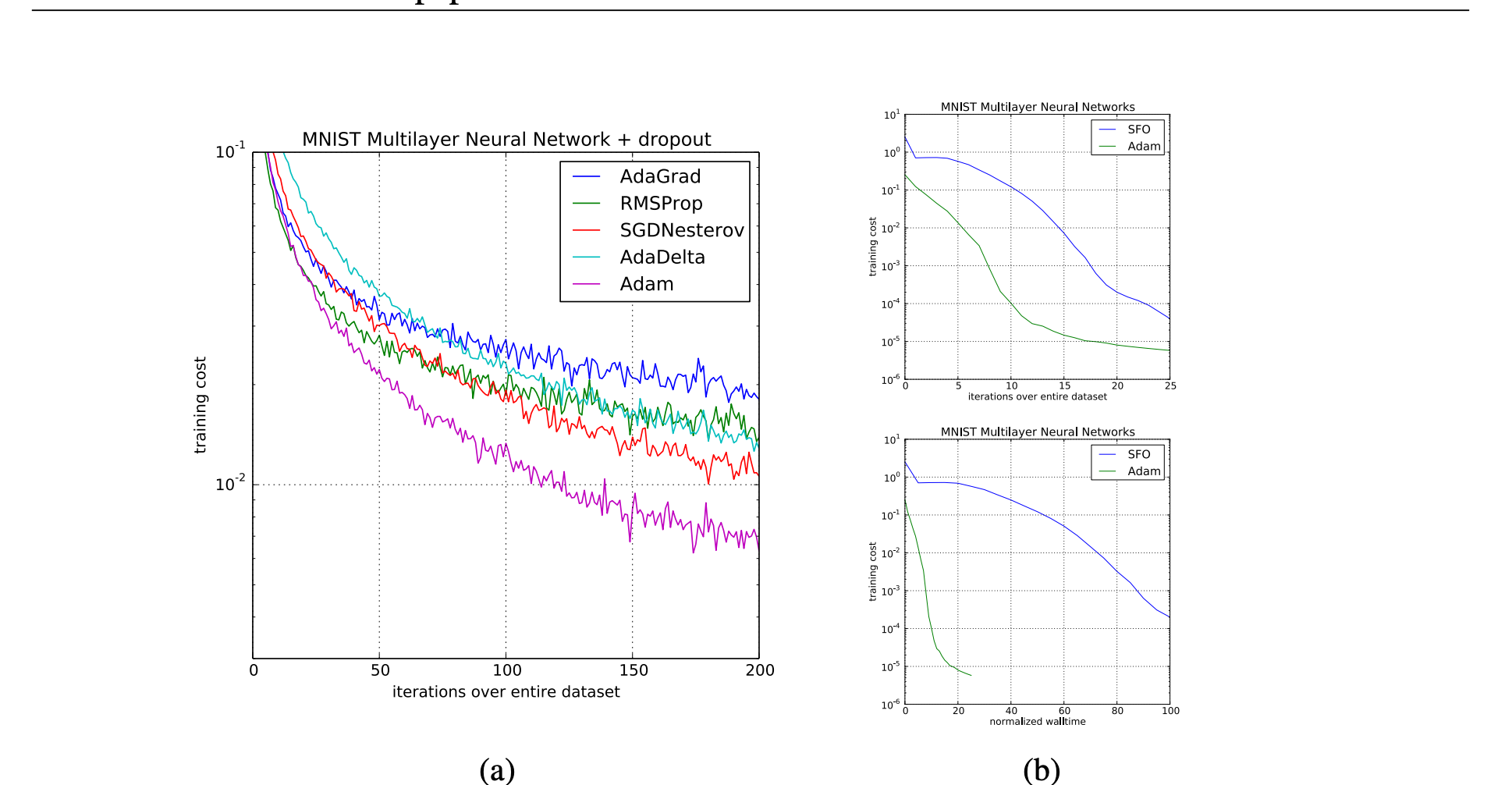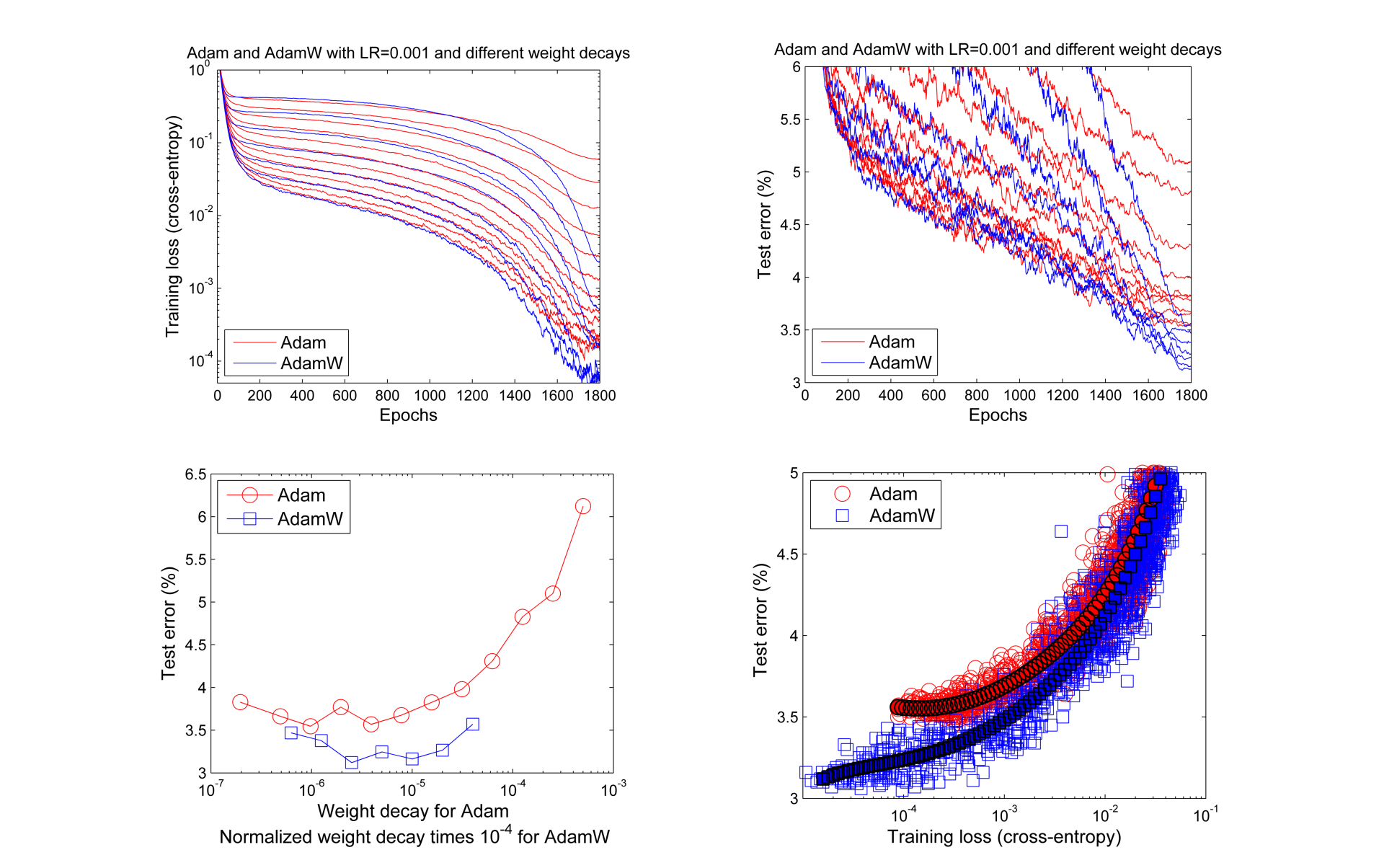# Optimizers in JAX and Flax

Optimizers are applied when training neural networks to reduce the error between the true and predicted values. This optimization is done via gradient descent. Gradient descent adjusts errors in the network through a cost function. In JAX, optimizers are applied from the Optax library.

Optimizers can be classified into two broad categories:

• Accelerated stochastic gradient descent (SGD), for example, SGD with momentum, heavy-ball method (HB), and Nesterov accelerated gradient (NAG).

Let's look at common optimizer functions used in JAX and Flax.

When performing optimization, adaptive optimizers start with large update steps but reduce the step size as they get close to the global minimum. This ensures that they don't miss the global minimum.

Adaptive optimizers such as Adam are quite common because they converge faster, but they may have poor generalization.

SGD-based optimizers apply a global learning rate on all parameters, while adaptive optimizers calculate a learning rate for each parameter.

The authors of AdaBelief introduced the optimizer to:

• Converge fast as in adaptive methods.
• Have good generalization like SGD.
• Be stable during training.

AdaBelief works on the concept of "belief" in the current gradient direction. If it results in good performance, then that direction is trusted, and large updates are applied. Otherwise, it's distrusted and the step size is reduced.

Let's look at a Flax training state that applies the AdaBelief optimizer.

``````from flax.training import train_state

def create_train_state(rng, learning_rate):
"""Creates initial `TrainState`."""
cnn = CNN()
params = cnn.init(rng, jnp.ones([1, size_image, size_image, 3]))['params']
return train_state.TrainState.create(
apply_fn=cnn.apply, params=params, tx=tx)``````

Here's the performance of AdaBelief on various tasks as provided by its authors.

``````from flax.training import train_state

def create_train_state(rng, learning_rate):
"""Creates initial `TrainState`."""
cnn = CNN()
params = cnn.init(rng, jnp.ones([1, size_image, size_image, 3]))['params']
return train_state.TrainState.create(
apply_fn=cnn.apply, params=params, tx=tx)``````

Adam is a common optimizer in deep learning because it gives good results with default parameters, is computationally inexpensive, and uses little memory.

``````from flax.training import train_state

def create_train_state(rng):
"""Creates initial `TrainState`."""
model = LSTMModel()
return train_state.TrainState.create(
apply_fn=model.apply, params=params, tx=tx)``````Training of multilayer neural networks on MNIST images. (a) Neural networks using dropout stochastic regularization. (b) Neural networks with deterministic cost function

AdamW is Adam with weight decay regularization. Weight decay regularization penalizes the cost function making the weights smaller during backpropagarion. It results in small weights that lead to better generalization. In some cases, Adam with decoupled weight decay leads to better results compared Adam with L2 regularization.

``````from flax.training import train_state

def create_train_state(rng):
"""Creates initial `TrainState`."""
model = LSTMModel()
return train_state.TrainState.create(
apply_fn=model.apply, params=params, tx=tx)``````Learning curves (top row) and generalization results (bottom row) obtained by a 26 2x96d ResNet trained with Adam and AdamW on CIFAR-10

RAdam aims to solve large variances during the early training stages when applying an adaptive learning rate.

``````from flax.training import train_state

def create_train_state(rng, learning_rate):
"""Creates initial `TrainState`."""
cnn = CNN()
params = cnn.init(rng, jnp.ones([1, size_image, size_image, 3]))['params']
return train_state.TrainState.create(
apply_fn=cnn.apply, params=params, tx=tx)``````

AdaFactor is used for training large neural networks because it is implemented to reduce memory utilization.

``````from flax.training import train_state

def create_train_state(rng, learning_rate):
"""Creates initial `TrainState`."""
cnn = CNN()
params = cnn.init(rng, jnp.ones([1, size_image, size_image, 3]))['params']
return train_state.TrainState.create(
apply_fn=cnn.apply, params=params, tx=tx)``````

### Fromage

Fromage introduces a distance function on deep neural networks called deep relative trust. It requires little to no learning rate tuning.

``````from flax.training import train_state

def create_train_state(rng, learning_rate):
"""Creates initial `TrainState`."""
cnn = CNN()
params = cnn.init(rng, jnp.ones([1, size_image, size_image, 3]))['params']
tx = optax.fromage(learning_rate)
return train_state.TrainState.create(
apply_fn=cnn.apply, params=params, tx=tx)``````

### Lamb – Layerwise adaptive large batch optimization

Lamb aims to enable the training of deep neural networks by computing gradients using large mini-batches. It leads to good performance on attention-based models such as Transformers and ResNet-50.

Subscribe